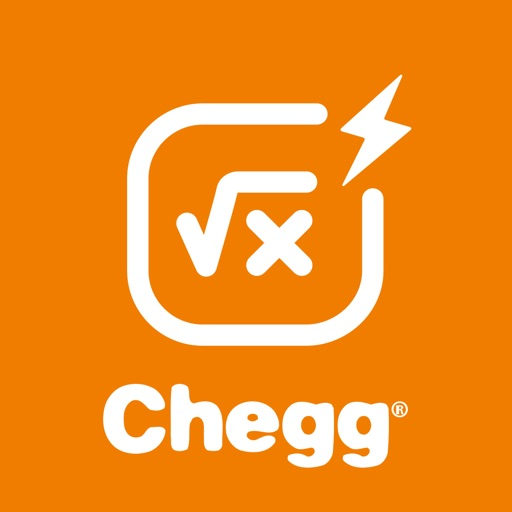Chegg Math Solver - math help# Chegg Math Solver - math help

## Snap a photo math solver 7/24

1.20.0 for iPhone
Free
7.9

582 Ratings

Size

66.2 MB

Chegg Math Solver - math help Description
Fear no math problem with the Chegg Math Solver app. Need homework help? Stuck on a hard problem? Prepping for a test? Use our guided, step-by-step math solver to get more homework help than a calculator can provide. Chegg Math Solver is more than a calculator. It digs into each step of your algebra, pre-calculus, or calculus math problems to learn how and why they were solved that way.

It’s easy to input a math problem in Chegg Math Solver with the photo math transform feature. You simply need to scan a math equation and take a photo with the camera of your mobile device. Chegg Math Solver will transform your photo into math equation and show you the step-by-step solution in a few seconds. The photo math magic saves your efforts to input complicated math problems from the calculator.

• Understand the how and why of your math solutions. With our math solver, you’ll see how to tackle each step — making it easy to fully understand how to approach it for next time. Use the photo math feature to easily snap a pic of your equation and get started.

• Learn from detailed step-by-step explanations. More than a calculator, our math solver walks you through each part of the solution, so you can get the homework help you need and learn how each step leads you to the right answer.

• Dig deeper into specific steps. Our math solver does what a calculator won’t. It can break down steps into smaller sub-steps, so you can dig deeper into the problem and see why it’s solved that way. It’s easy.

• Chegg Math Solver is for every student. No matter you are struggling with your math homework, preparing for your math final exams, or just want to understand math problems in depth, start Chegg Math Solver today and try the photo math transform function. Chegg Math Solver is the trusted math help to make math easier.

• Chegg Math Solver is for every parent who want to help your children learning math. It explains the solution of complicated math problems step by step, and makes them easier to understand. Start Chegg Math Solver today to help your children get better grades.

Leverage the photo math magic of Chegg Math Solver to help with your schoolwork in:
• Pre-algebra: fractions, linear equations, negative numbers, linear inequalities, powers and roots
• Pre-calculus: exponential and logarithmic functions, adding matrices, multiplying matrices, matrix inverses and determinants
• Calculus: fundamental derivatives, general derivatives, curve sketching, fundamental integrals, general integrals
• Linear algebra: matrix operations, inverse matrices, determinants, characteristic polynomial, eigenvalues

Chegg Math Monthly Subscription
When subscribing to Chegg Math, you will be charged automatically to your Apple ID account. Your subscription will then automatically renew each month unless canceled at least 24 hours before the end of the current period. (Account is charged for renewal up to 24 hours before the end of the current period.) You can manage your subscriptions and turn off auto-renewal in your App Store account settings after purchase.

Feedback and bug reports: appfeedback@chegg.com
Chegg Math Solver - math help 1.20.0 Update
2020-10-11 Version History
You spoke, we listened. We’ve fixed some bugs and improved usability with this version.
More
Price:
Free
Version:
1.20.0
Size:
66.2 MB
Genre:
Education
Update Date:
2020-10-11
Developer:
Chegg, Inc.
Language:
English
More